# Electronics and Communication Engineering - Exam Questions Papers

26.

The figure below shows a long infinite length conduction placed as shown.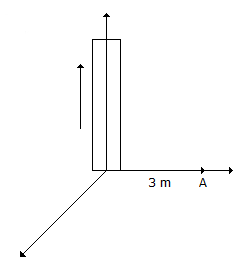Current flowing in a conductor is 2 amps. Then magnetic field at point A is:

 A.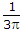B.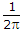C.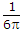D. No magnetic field

Answer: Option C

Explanation:

No answer description available for this question. Let us discuss.

27.

Consider the following statements regarding the class-B power amplifiers (Complementary symmetry type):

1. The efficiency of the amplifier is higher than that of class-A amplifier
2. The power output is low
3. Crossover distortion is present
4. The standby power dissipation is absent.
Which of the above statements are correct?

 A. 1, 2 and 3 B. 1, 2 and 4 C. 1, 3 and 4 D. 2, 3 and 4

Answer: Option C

Explanation:

No answer description available for this question. Let us discuss.

28.

Consider the following statements:
For a 10 m long common power line connecting a switch to a light bulb

1. It is a distributed circuit.
2. Time delay for propagation through it is negligible.
3. It is the form of a shielded coaxial cable of circular cross-section.
4. As the intensity of the lamp varies, input impedance of this line also changes.
Which of the above statements is/are correct?

 A. 1 only B. 1 and 2 C. 2 and 3 D. 2 and 4

Answer: Option C

Explanation:

No answer description available for this question. Let us discuss.

29.

The equivalent of the block diagram in figure is given in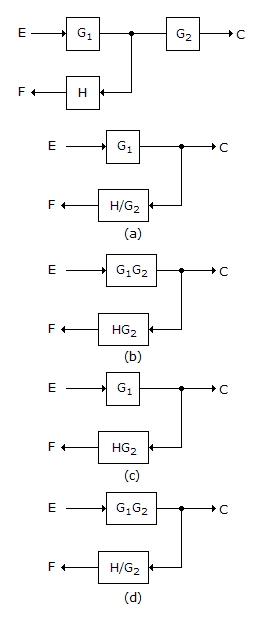A. Figure (a) B. Figure (b) C. Figure (c) D. Figure (d)

Answer: Option D

Explanation:

No answer description available for this question. Let us discuss.

30.

For a Hertz dipole antenna, the Half Power Beam Width (HPBW) in the E-plane is

 A. 360° B. 180° C. 90° D. 45°

Answer: Option B

Explanation:

No answer description available for this question. Let us discuss.

#### Current Affairs 2021

Interview Questions and Answers